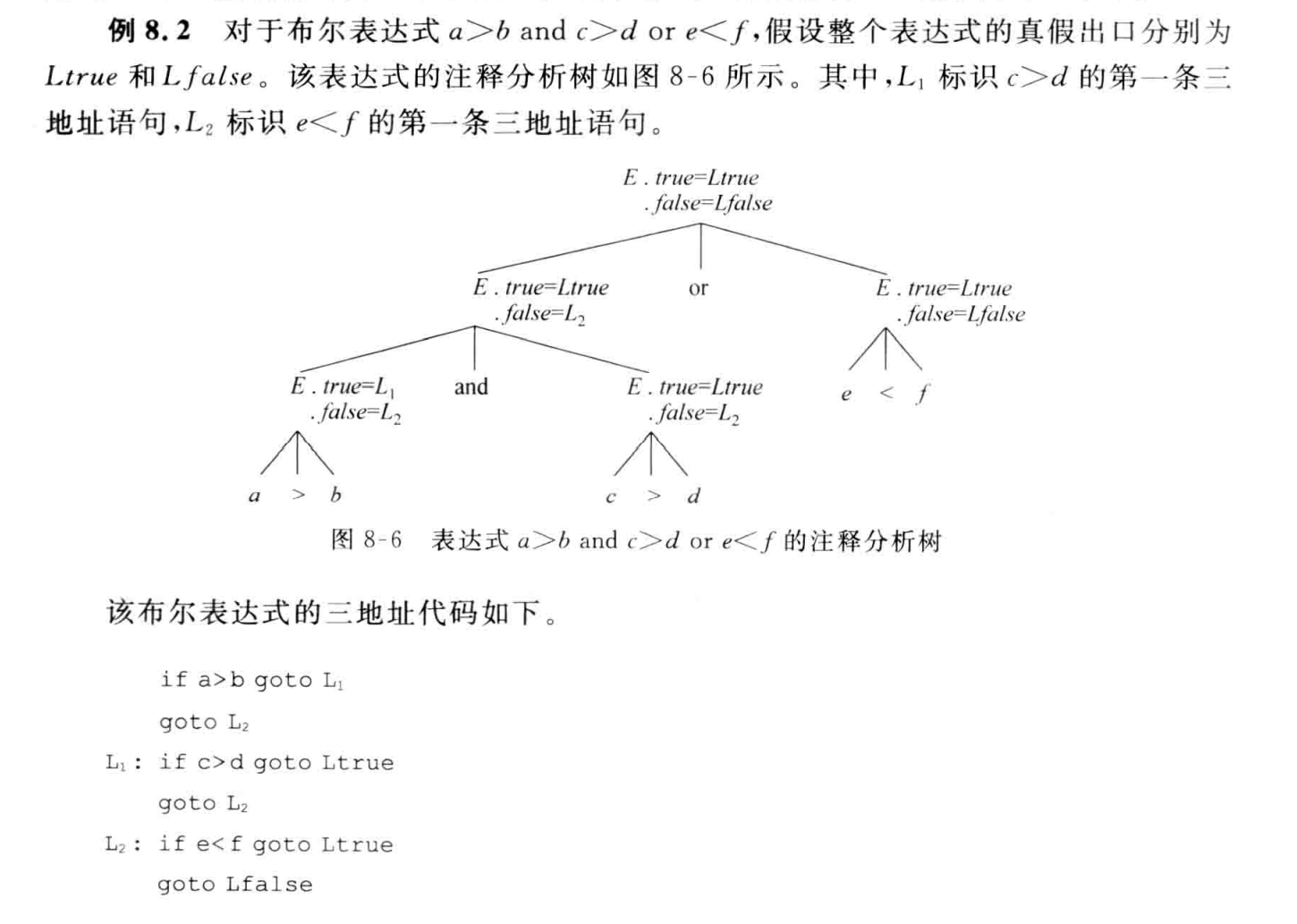## 语法分析

### 自顶向下分析方法

#### 构造文法的预测分析程序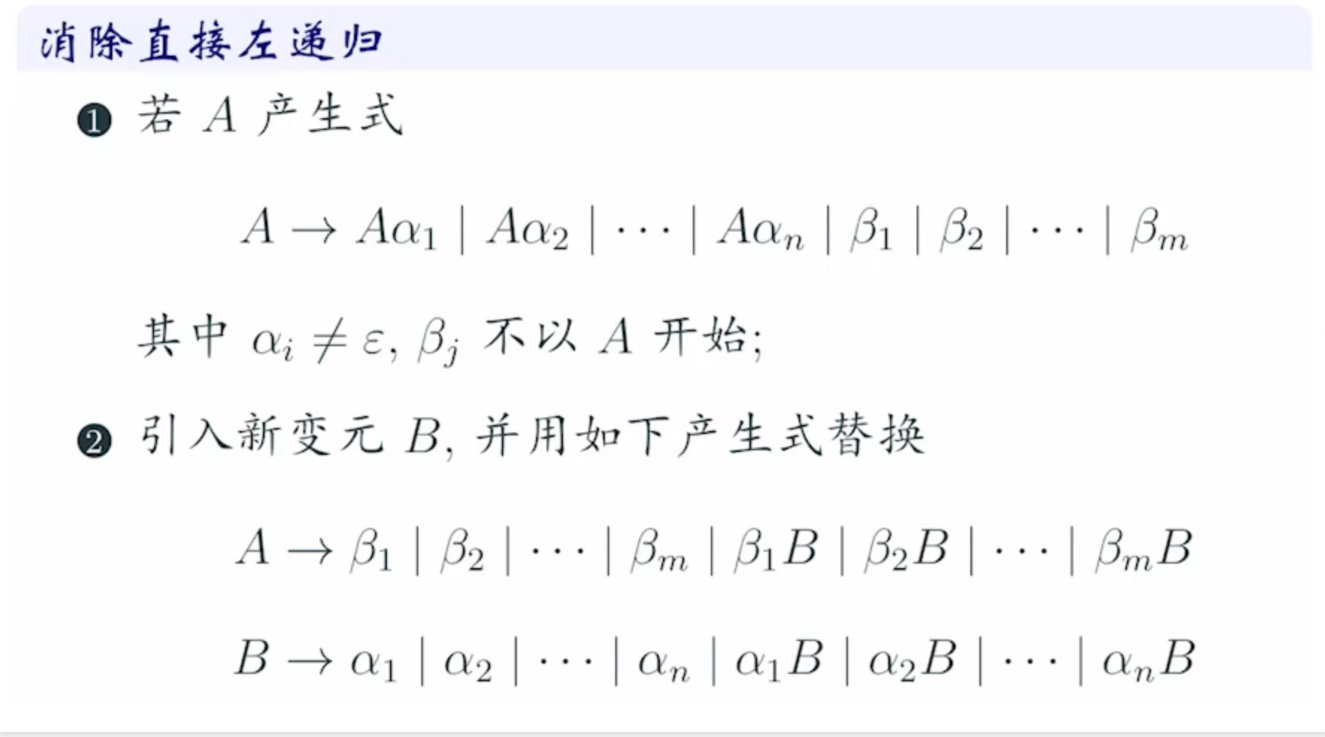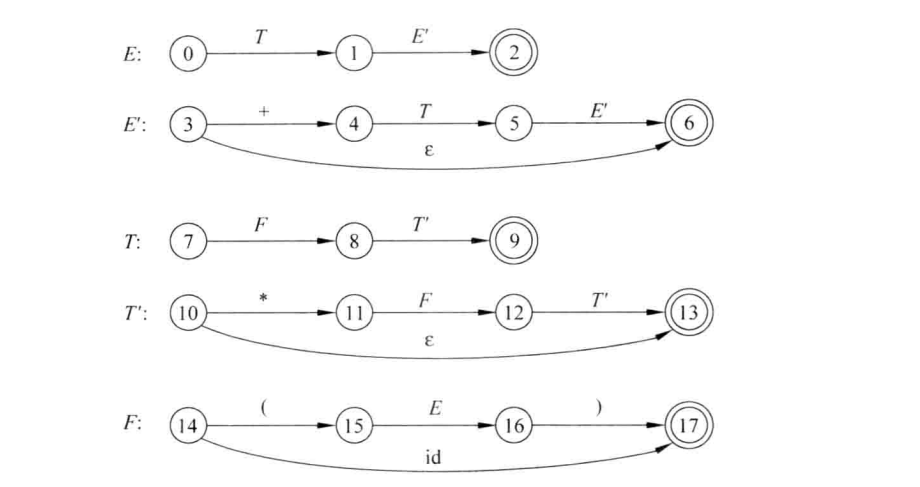真的很奇妙，不是吗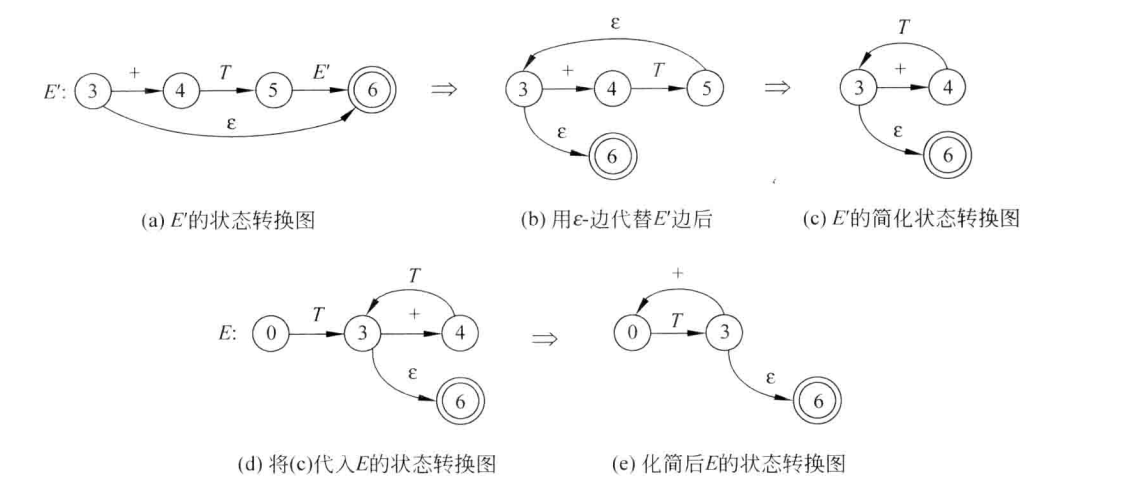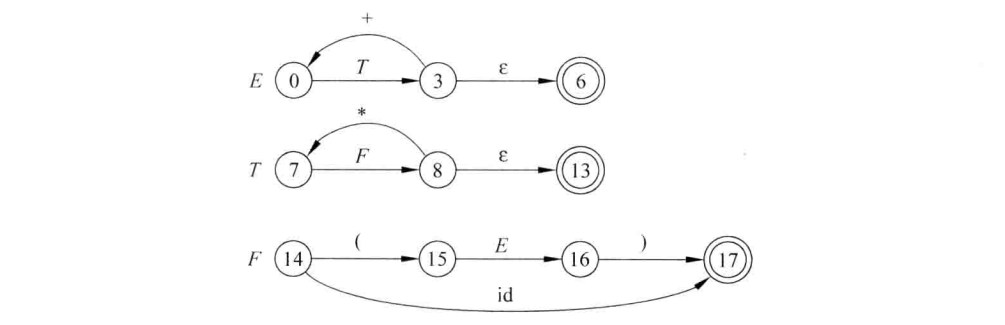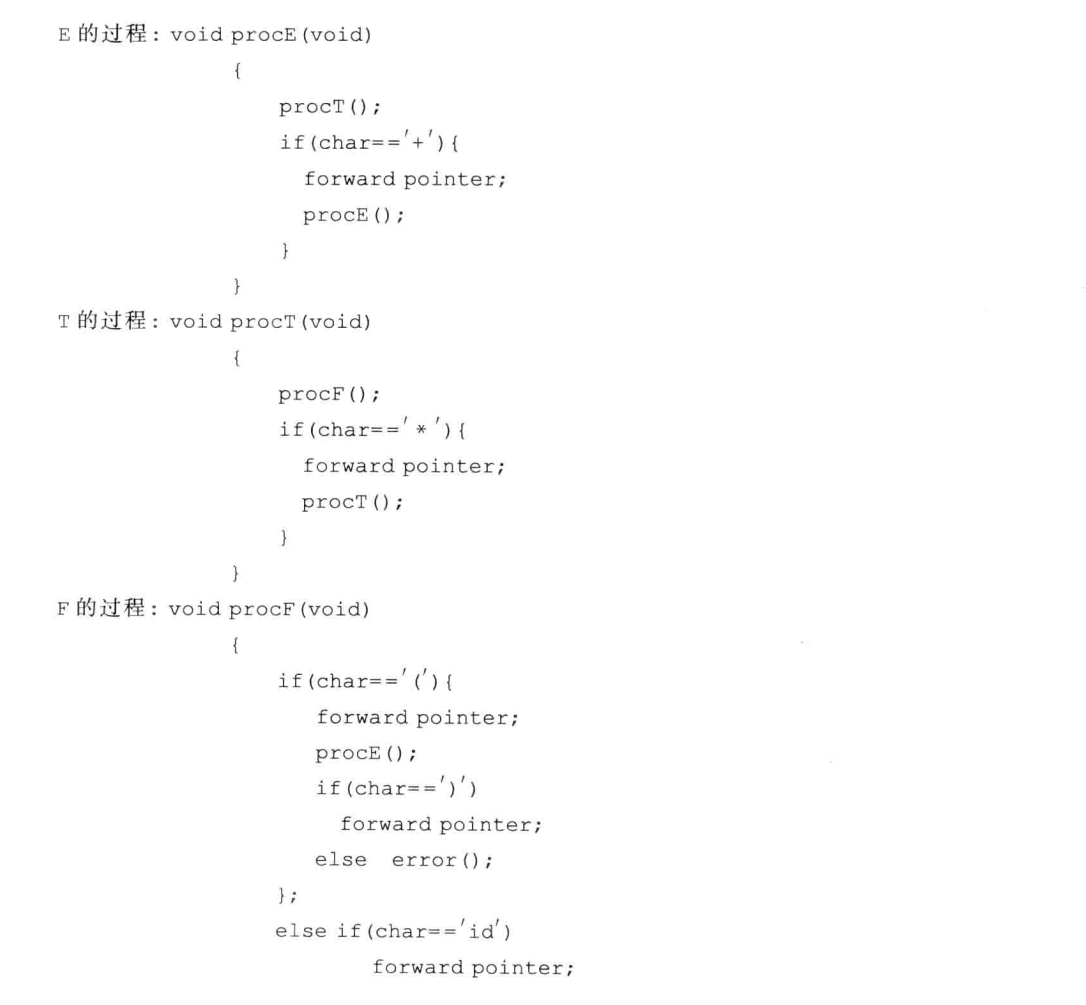#### 非递归预测分析

##### 预测分析表的构造
###### FIRST(X)

1. $X$为终结符，则$FIRST(X) = {X}$

2. $X$为非终结符，且有产生式$X→a…$，其中a为终结符，则$a∈FIRST(X)$

若有产生式$X→ε$，则$ε∈FIRST(X)$

3. 若有产生式$X→Y…$，且Y为非终结符，则将$FIRST(Y)$中的所有非$ε$元素加入$FIRST(X)$

若有产生式$X→Y_1 Y_2…Y_k$，如果对某个$i$，$FIRST(Y_1),FIRST(Y_2),…,FIRST(Y_{i-1})$都含有$ε$，则把$FIRST(Y_i)$中的所有非$ε$元素加入$FIRST(X)$中

若所有的$FIRST(Y_i)$均含有$ε$，则将$ε$加入$FIRST(X)$

###### FOLLOW(A)

2. 若有产生式$A→αBβ$，则把$FIRST(β)$中的所有非$ε$元素加入$FOLLOW(B)$
3. 若有产生式$A→αB$，或$A→αBβ$但$ε∈FIRST(β)$，则把$FOLLOW(A)$中的所有元素加入$FOLLOW(B)$
###### 构造预测分析表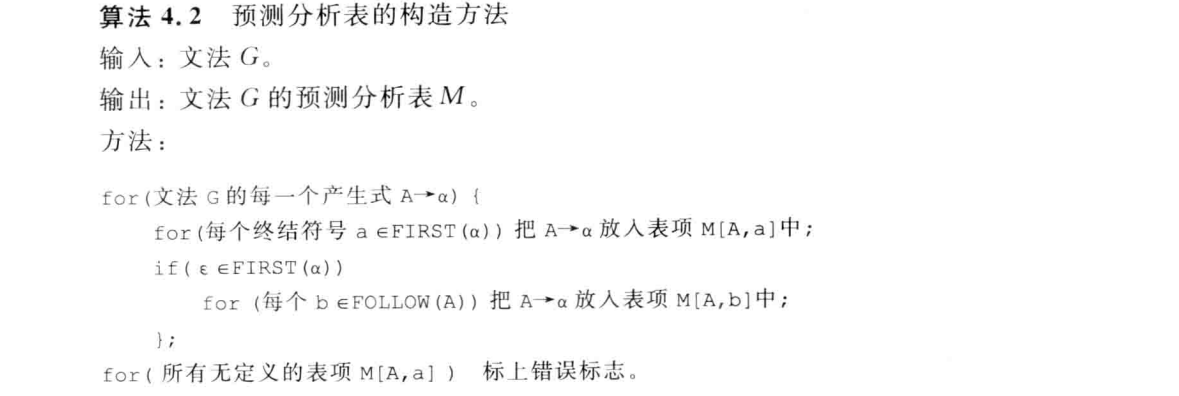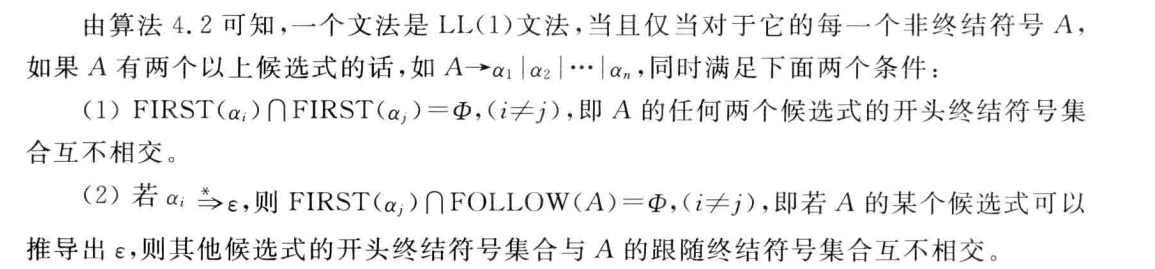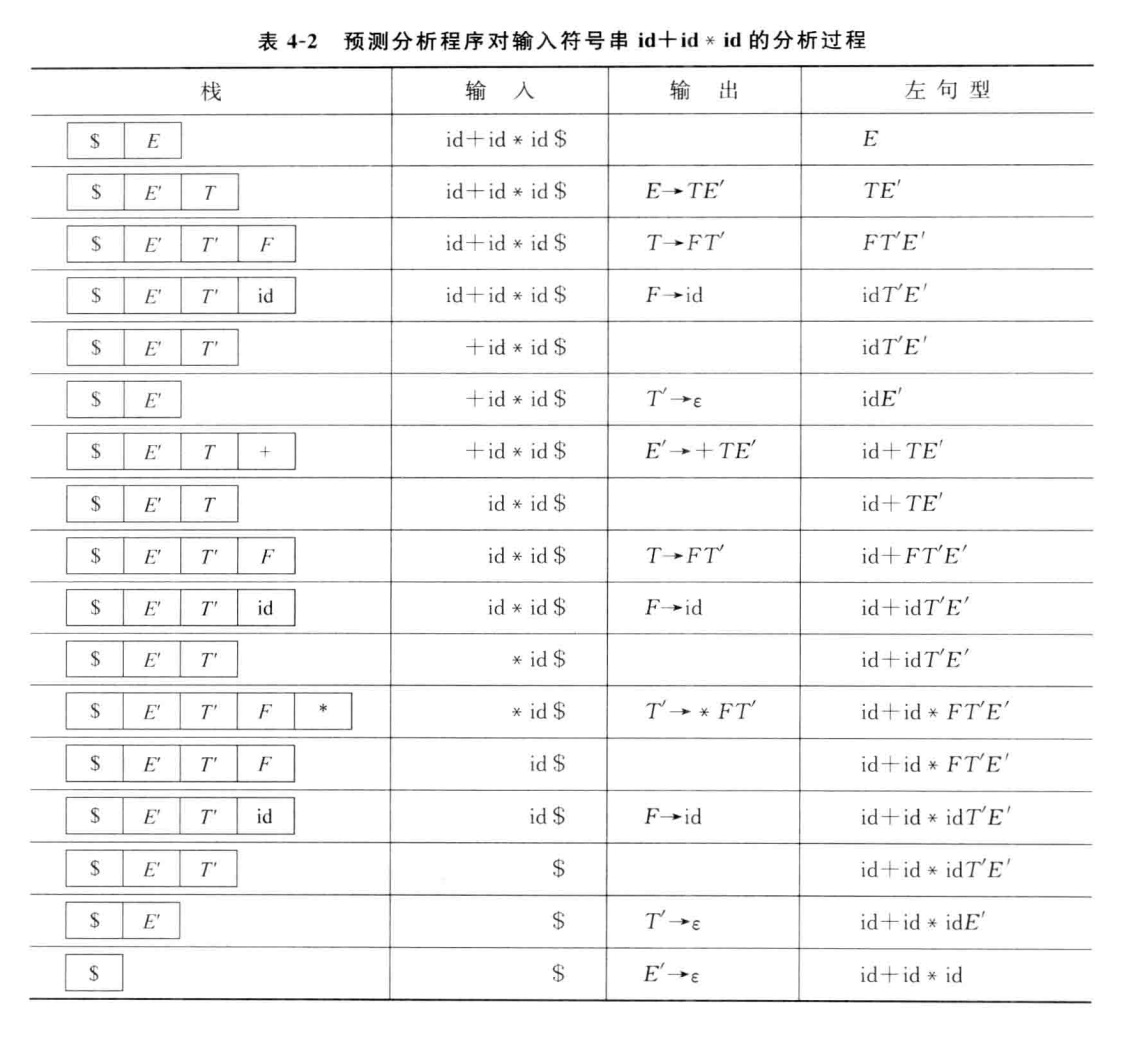##### 预测分析程序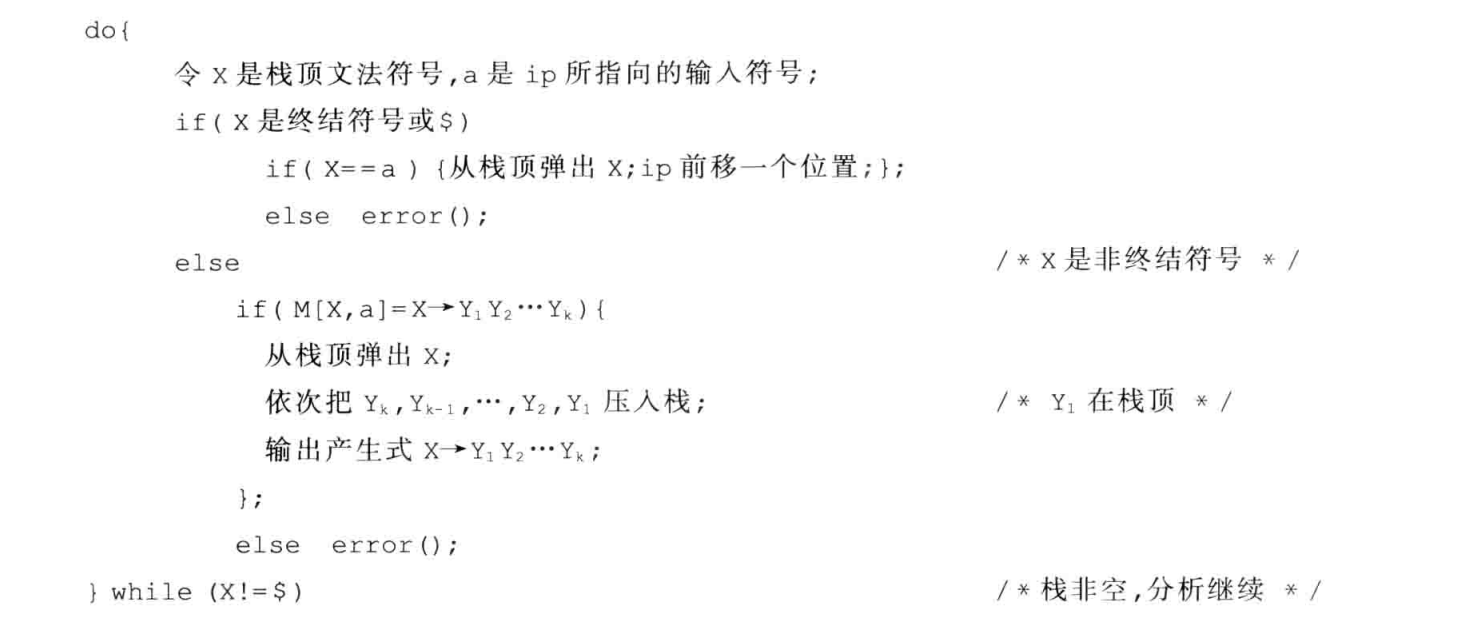### 自底向上分析方法

#### LR

##### 分析过程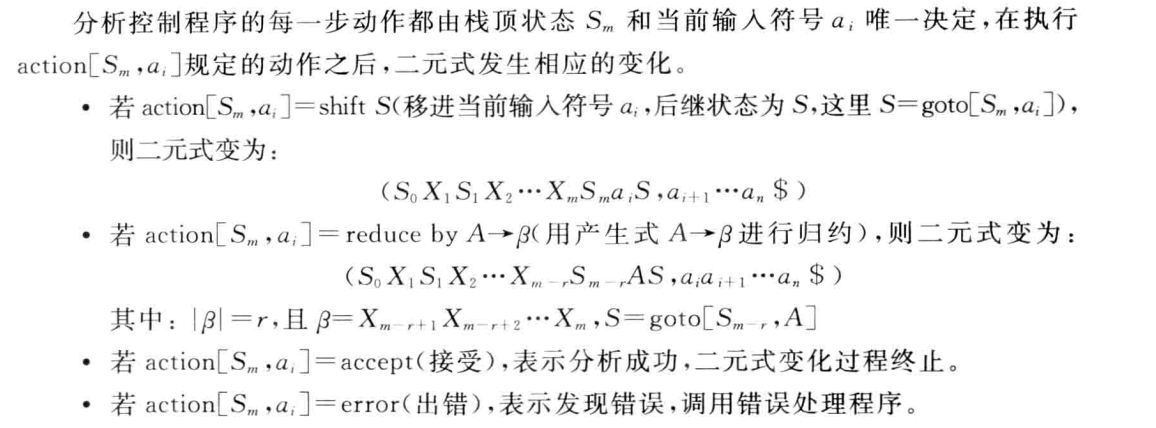##### SLR(1)分析表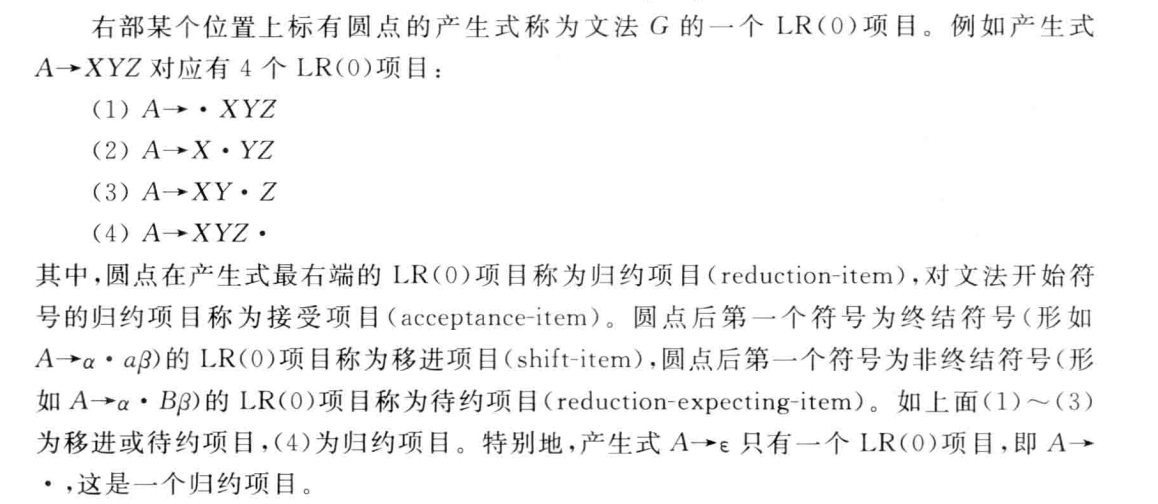###### 构造LR(0)项目集规范族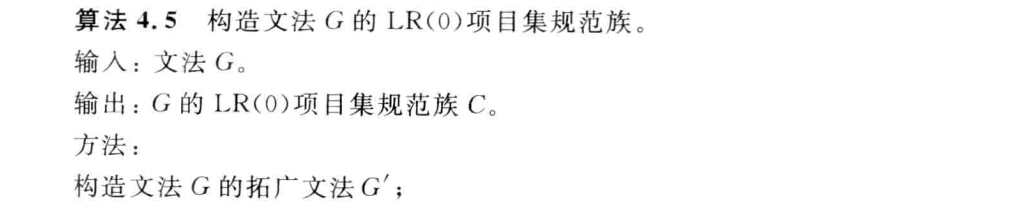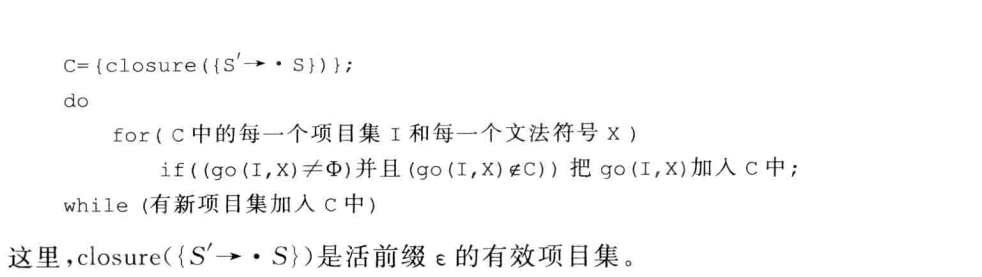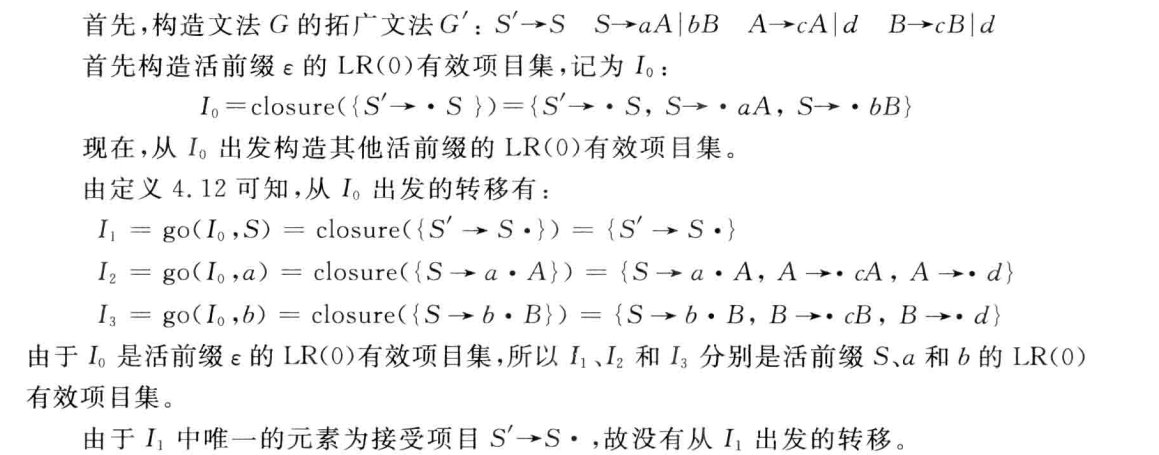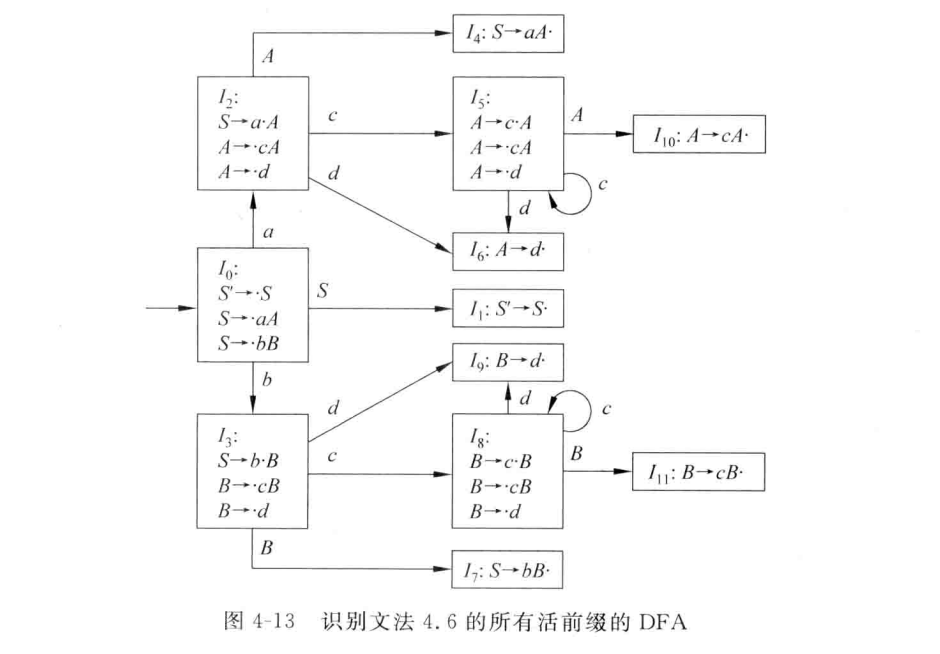###### 构造SLR(1)分析表

LR(0)可能会产生移进-规约冲突或者规约-规约冲突，SLR(1)考察非终结符号的FOLLOW集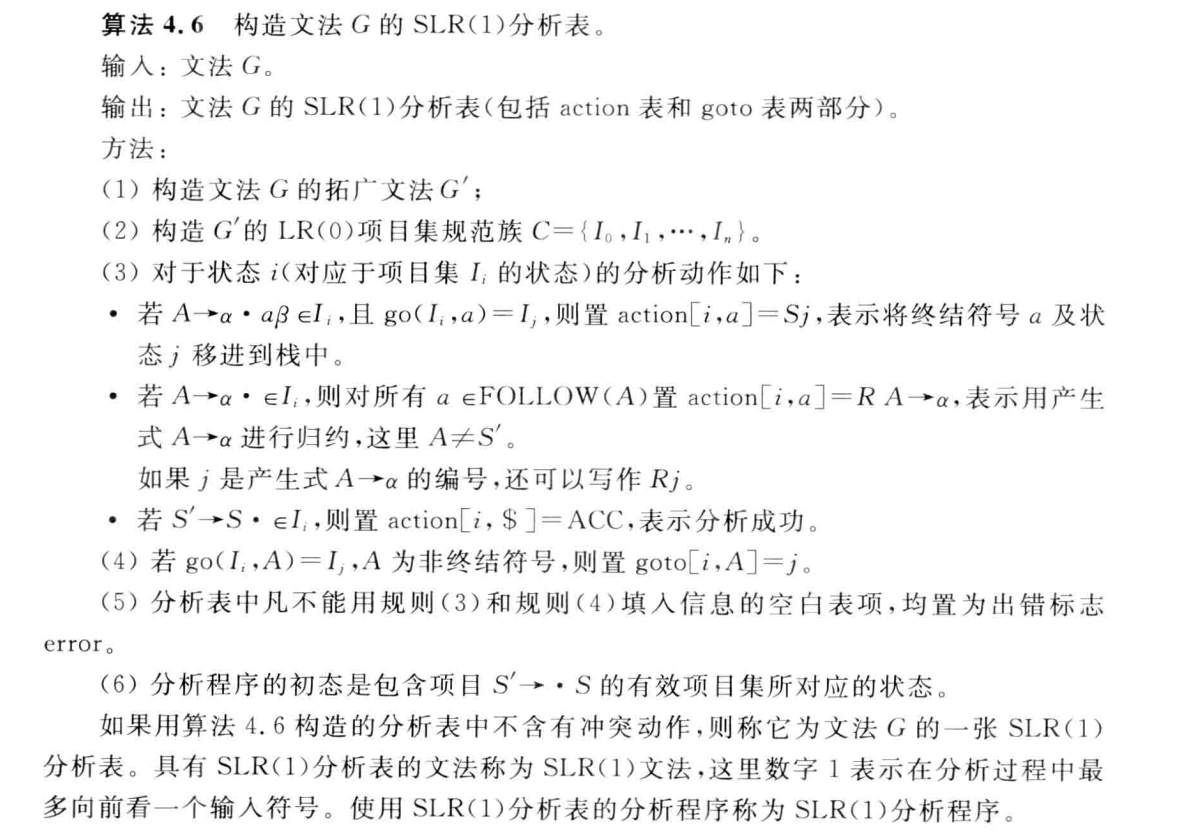##### LR(1)分析表

书上的概念看不懂，所以直接看例题。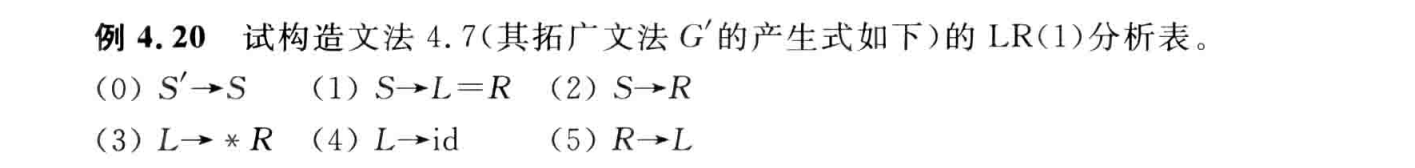$FIRST(βa)$ ，其中$β$为原式子该非终结符之后的剩余串，$a$为上个状态的展望符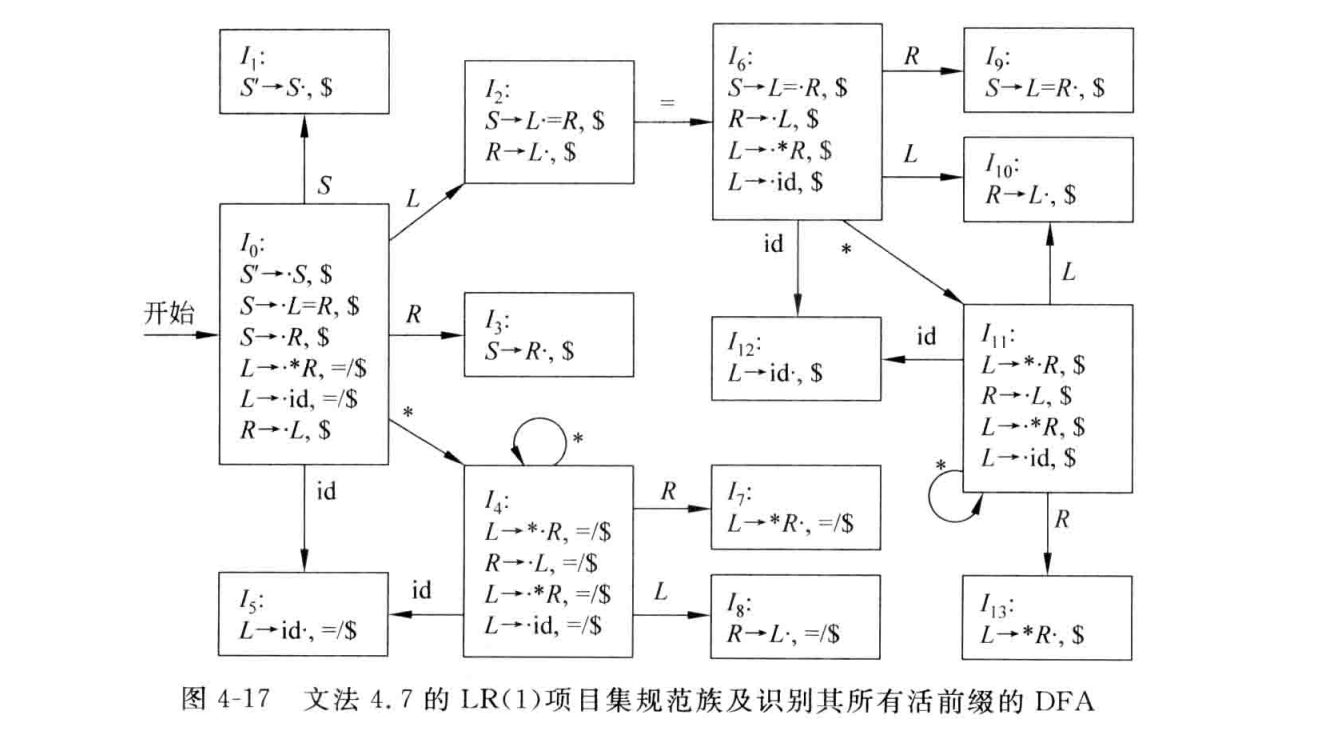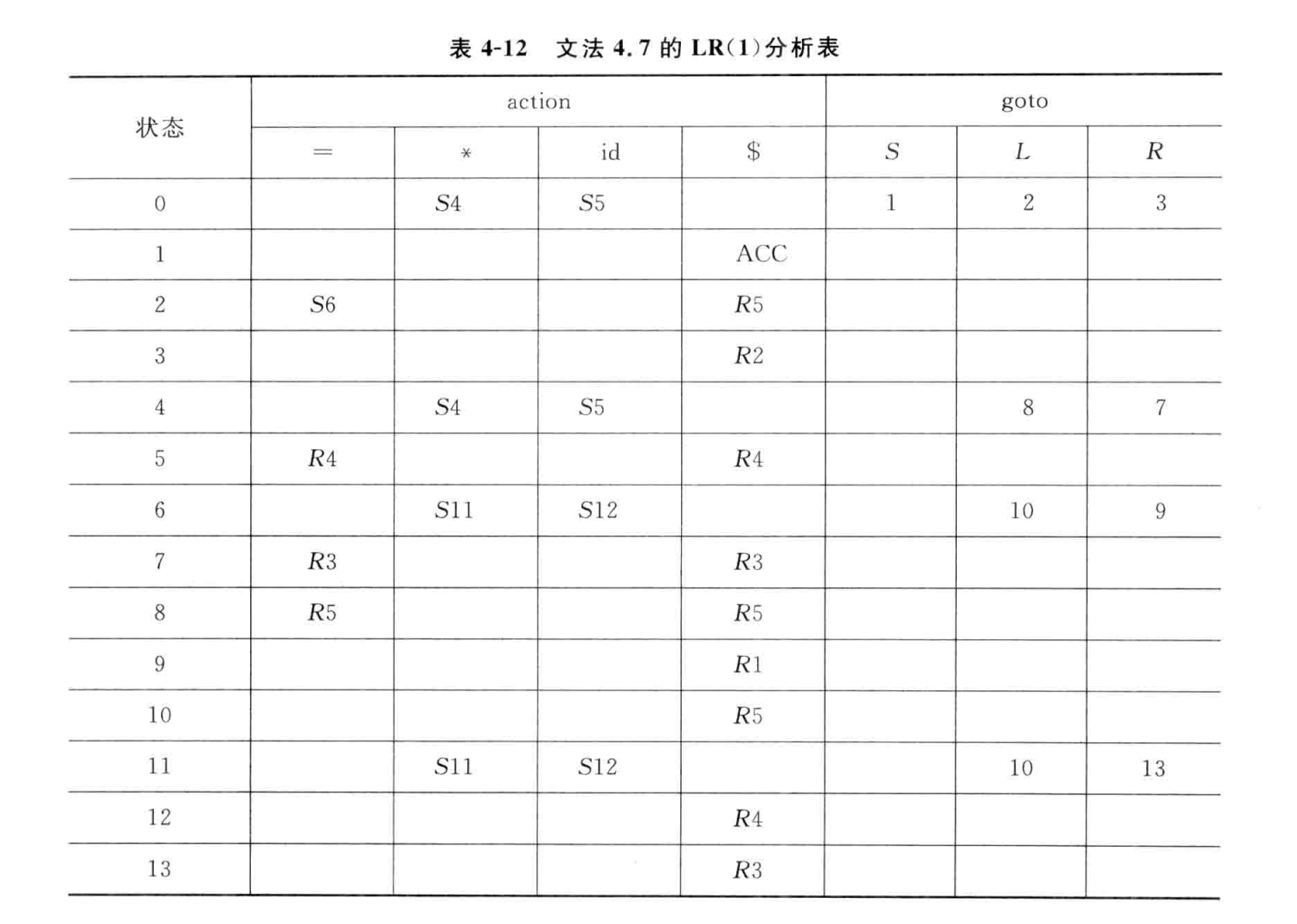##### LALR(1)分析表
###### 基本思想

①合并LR(1)项目集规范族中的同心集，以减少分析表的状态数

②用项目集的核代替项目集，以减少项目集所需的存储空间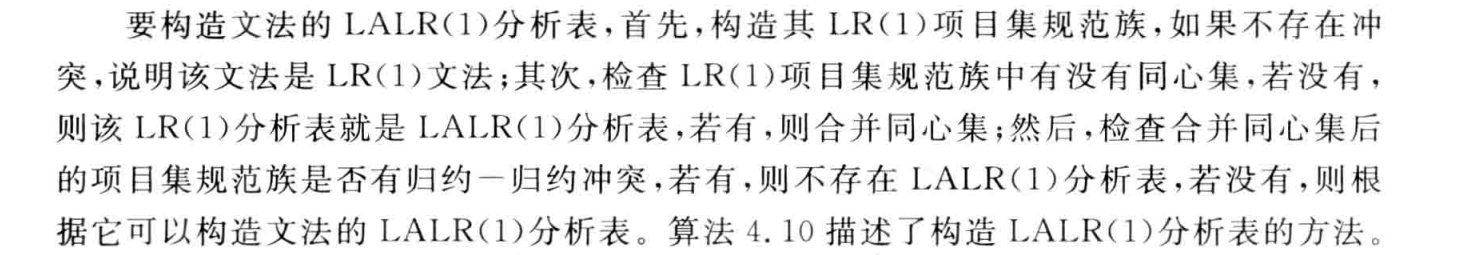##### 判断文法

###### $SLR(1)$ 和 $LR(1)$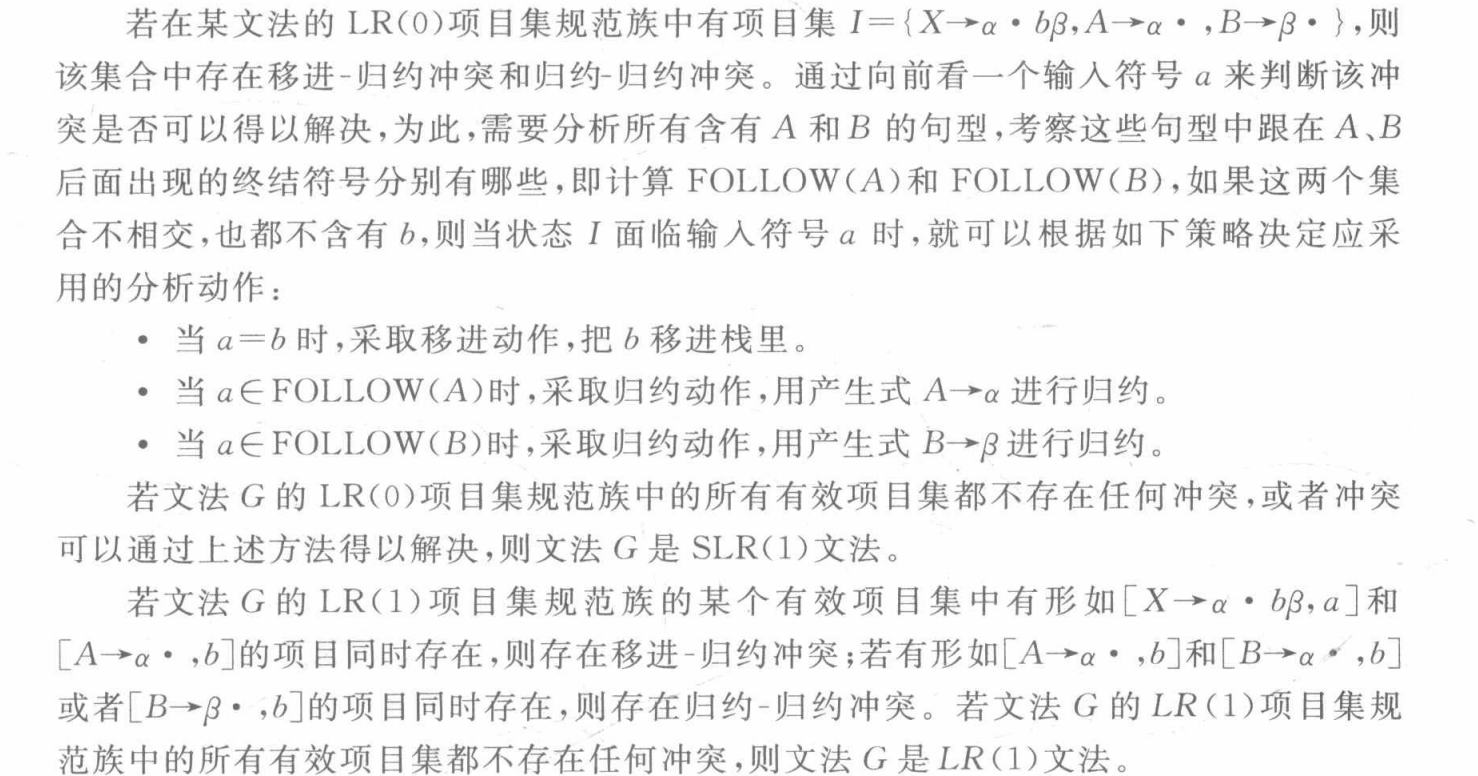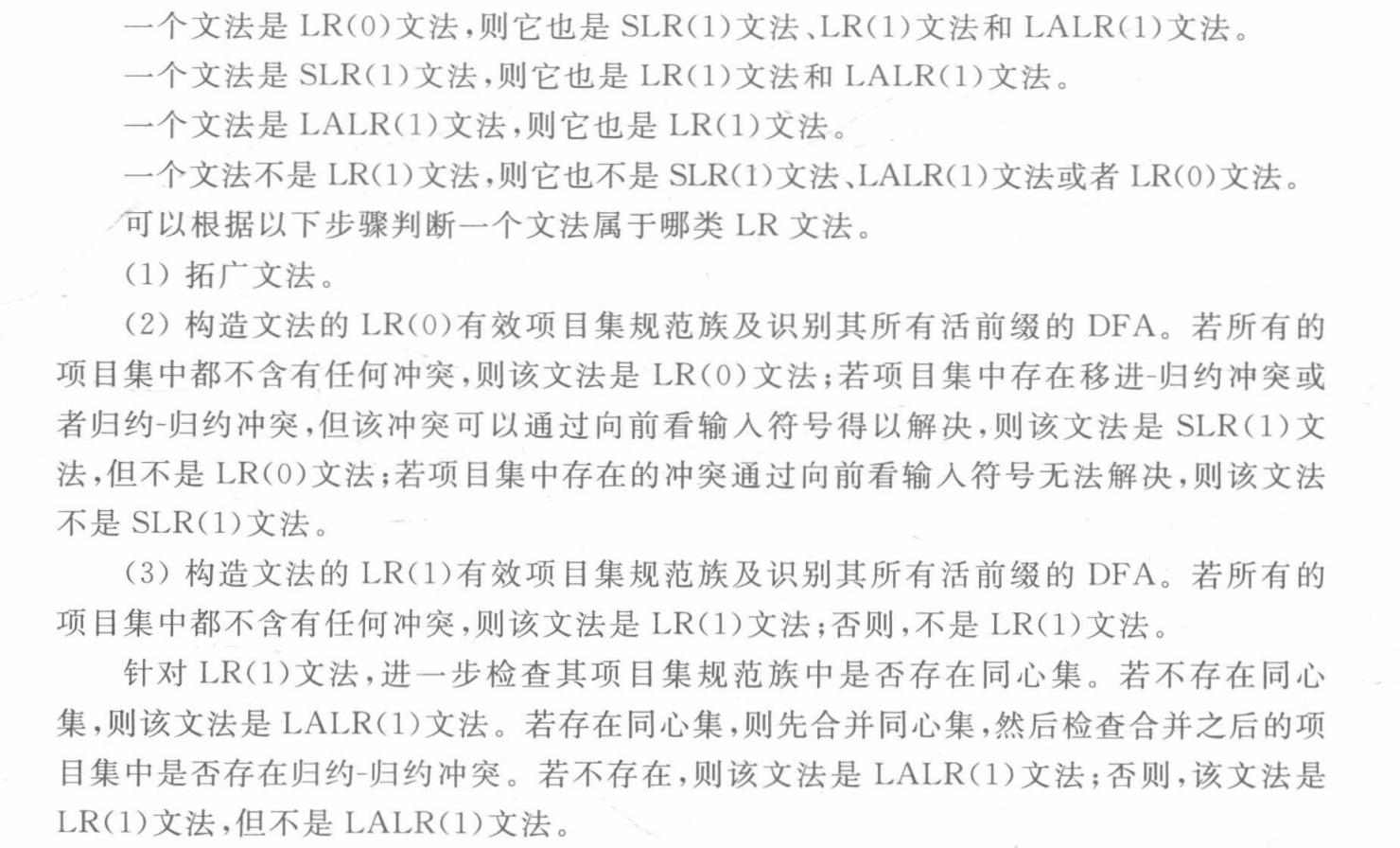## 语义分析

### 类型检查

#### 类型表达式

##### 数组

$array(I,T)$

a:array[1…10] of char;

##### 笛卡尔乘积

$T_1×T_2$

##### 记录

B : record

​ i : integer;

​ c : char;

​ r : real;

end;

B的类型表达式为record((i×integer)×(c×char)×(r×real))

struct B{

​ int i;

​ char c;

​ float r;

}

##### 指针

p: ↑row;

p的类型表达式为pointer(row)

##### 函数

function fun(a : char, b : integer): ↑integer;

fun的类型表达式为char × integer → pointer(integer)

#### 类型等价

##### C语言的类型等价

C语言对struct和union采用名字等价，其他采用结构等价。

## 运行环境

### 参数传递机制

建议看作业题7.2，结合答案的图看。

## 中间代码生成

### 中间代码形式

#### 三地址代码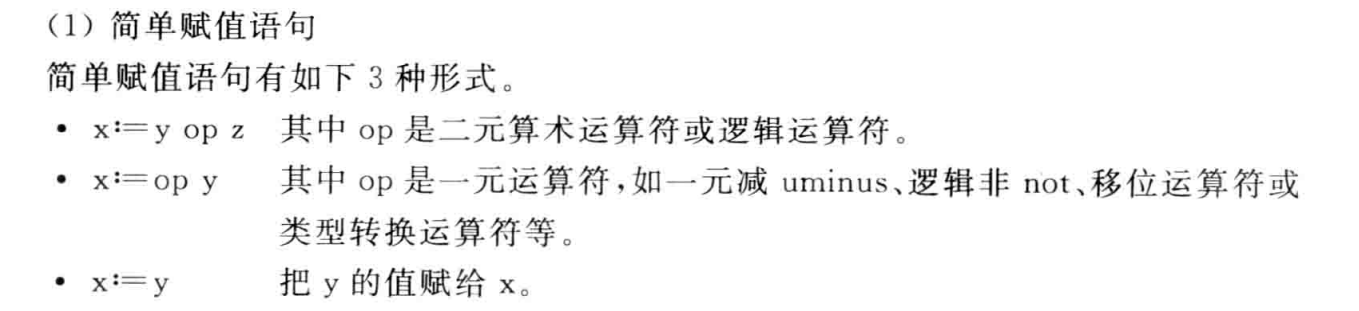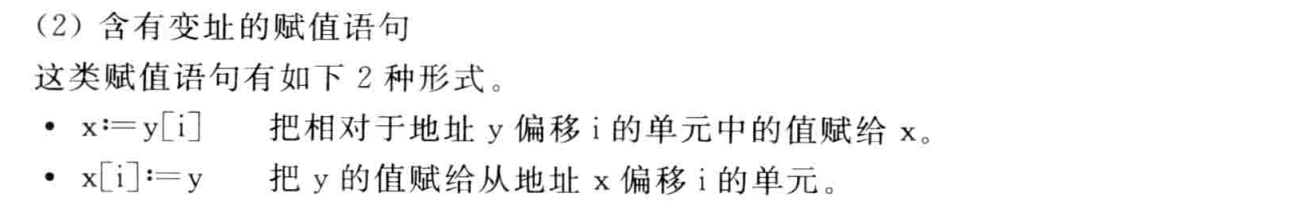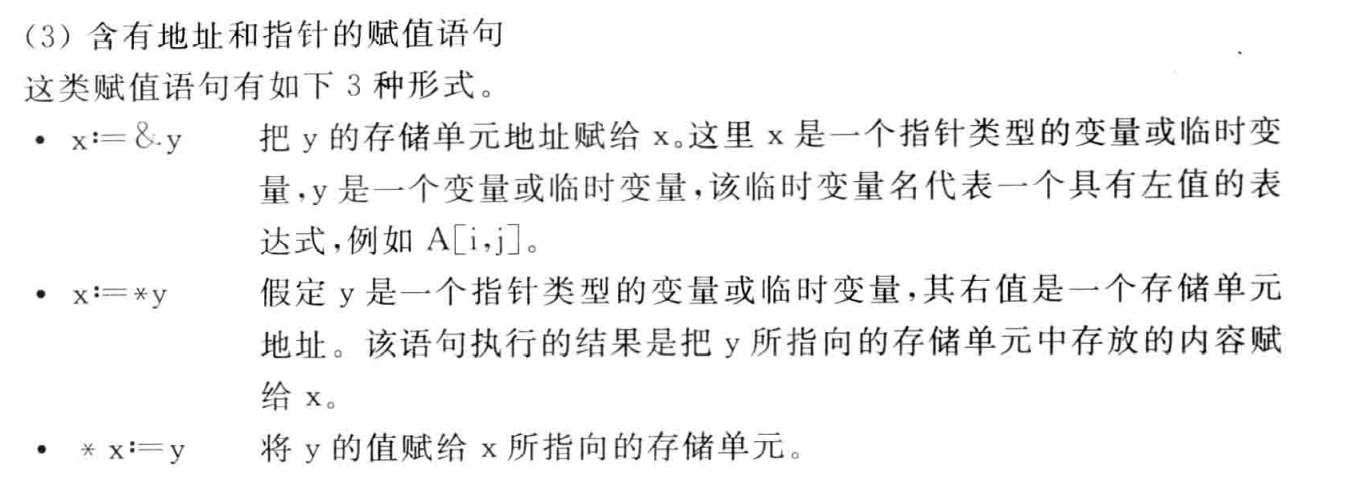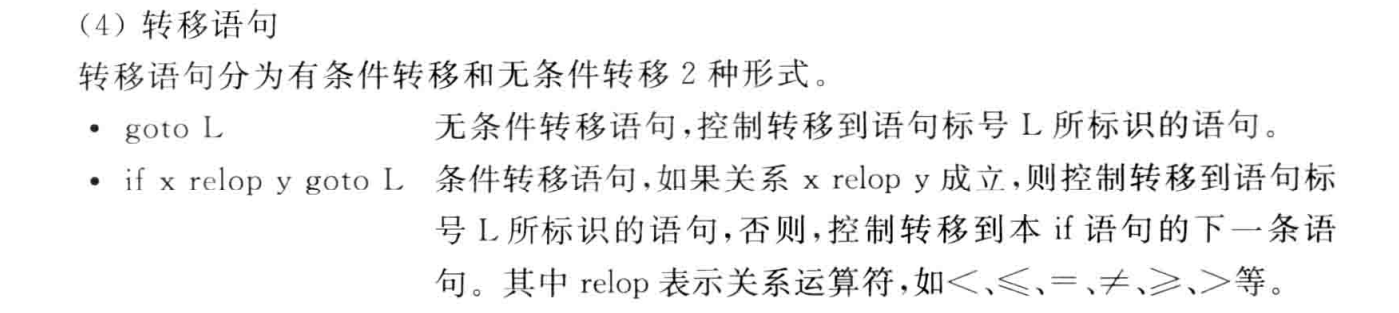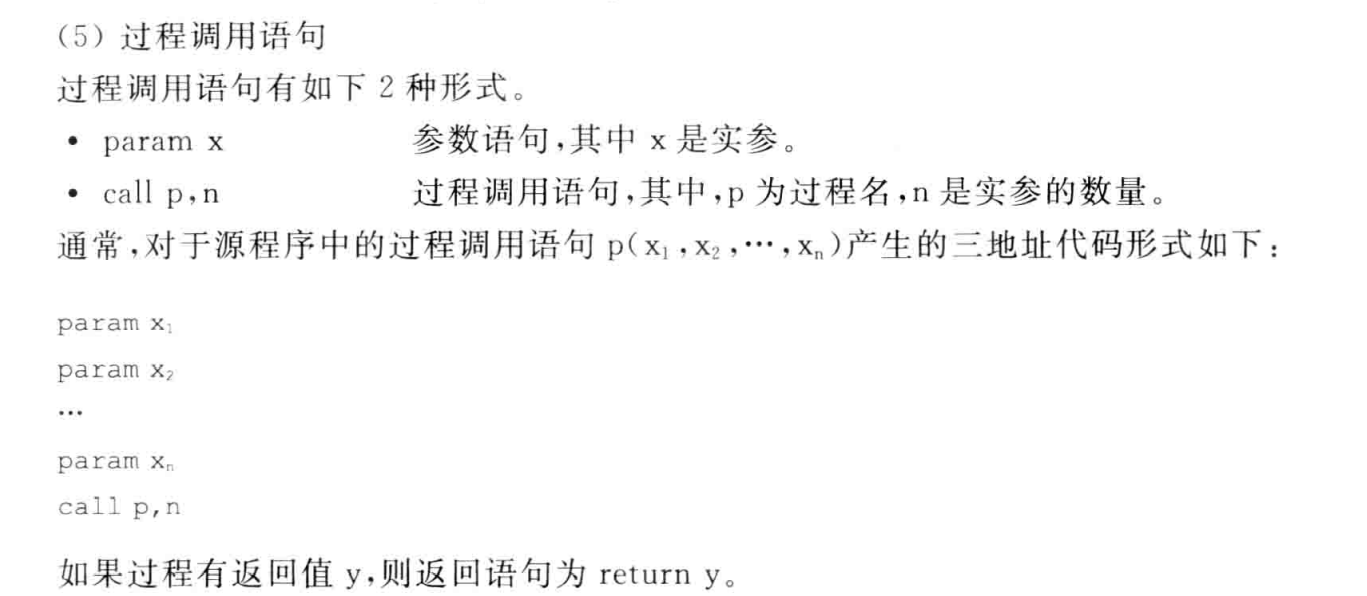#### 四元式

(op, arg1, arg2, result)

### 赋值语句的翻译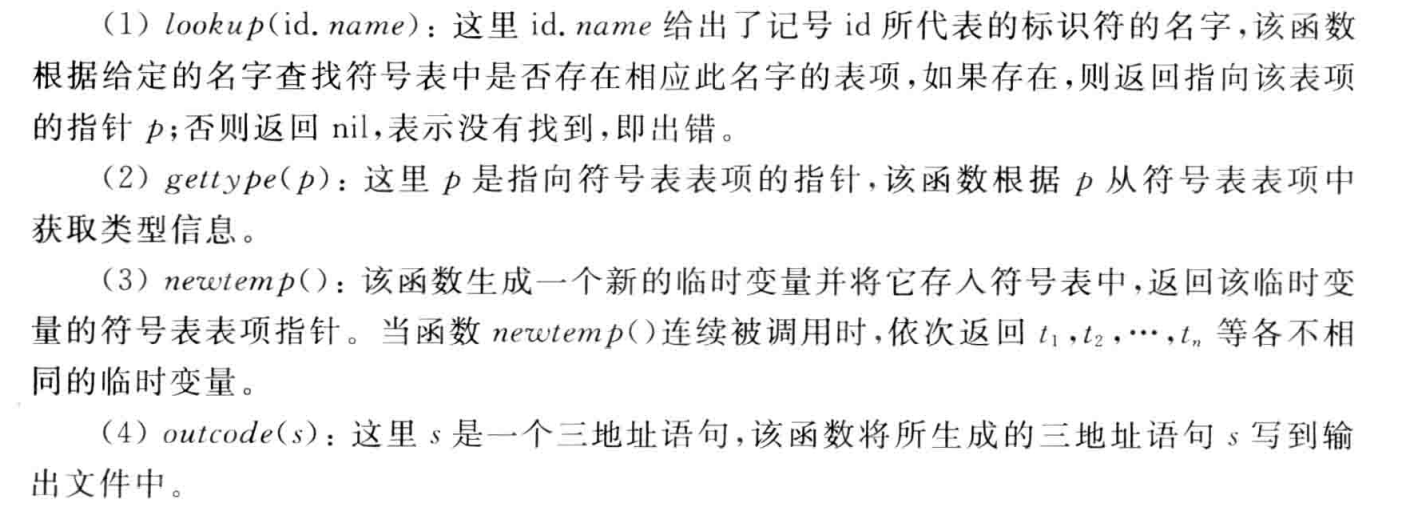#### 数组

##### 一维数组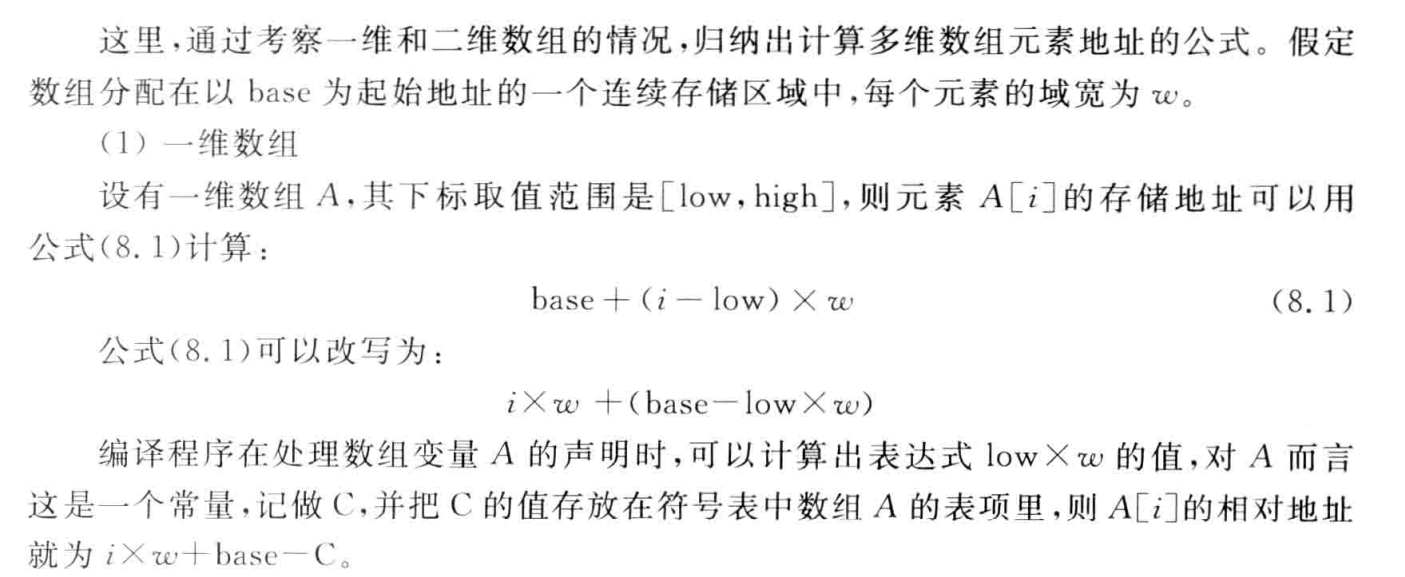##### 二维数组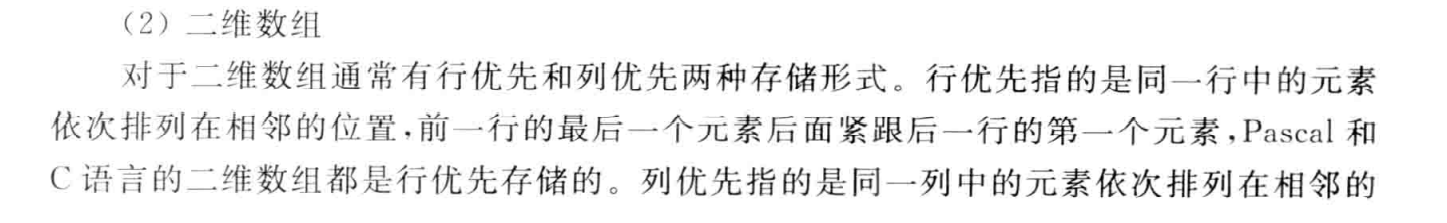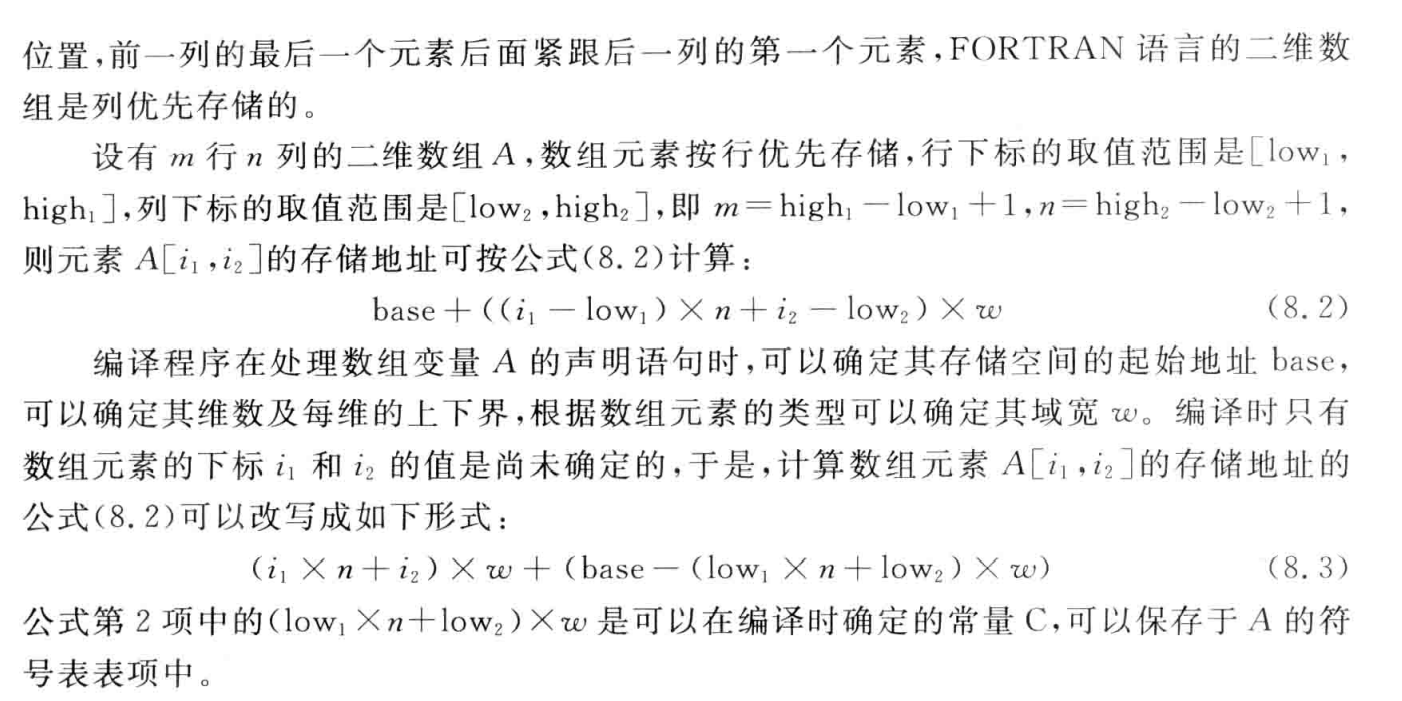### 布尔表达式的翻译

#### 控制流表示法